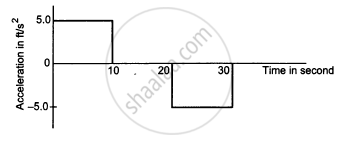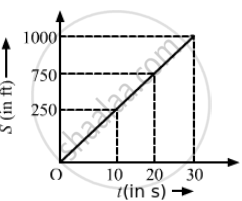Advertisement Remove all ads

# The acceleration of a cart started at t = 0, varies with time . Find the  distance travelled in 30 seconds and draw the position-time graph. - Physics

Short Note

The acceleration of a cart started at t = 0, varies with time as shown in the following figure. Find the distance travelled in 30 seconds and draw the position-time graph.Advertisement Remove all ads

#### Solution

In the first 10 seconds,

$S_1 = ut + \frac{1}{2}a t^2$

$= 0 + \frac{1}{2}5 \times {10}^2 = 250 ft$
At t = 10 s,
v = u + at = 0 + 5 × 10 = 50 ft/s
∴ From 10 to 20 seconds (∆t = 20 − 10 = 10 s), the particle moves with a uniform velocity of 50 ft/s.
Distance covered from t = 10 s to t = 20 s:
S2 = 50 × 10 = 500 ft

Between 20 s to 30 s, acceleration is constant, i.e., −5 ft/s2.
At 20 s, velocity is 50 ft/s.
t = 30 − 20 = 10 s
$S_3 = ut + \frac{1}{2}a t^2 = 50 \times 10 + \frac{1}{2}\left( - 5 \right) {10}^2$
$\Rightarrow S_3 = 500 - 250 = 250 ft$
otal distance travelled is 30 s:
S1 + S2 + S3
= 250 + 500 + 250
= 1000 ft

The position–time graph:Concept: Motion in a Straight Line - Position-time Graph
Is there an error in this question or solution?
Advertisement Remove all ads

#### APPEARS IN

HC Verma Class 11, 12 Concepts of Physics 1
Chapter 3 Rest and Motion: Kinematics
Q 7 | Page 51
Advertisement Remove all ads

#### Video TutorialsVIEW ALL 

Advertisement Remove all ads
Share
Notifications

View all notifications

Forgot password?